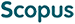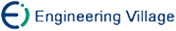杨季文, 李哲硕, 何书萍, 鲜 敏. 胞腔同调边缘学习算法研究[J]. 计算机研究与发展, 2013, 50(5): 1005-1011.
 引用本文: 杨季文, 李哲硕, 何书萍, 鲜 敏. 胞腔同调边缘学习算法研究[J]. 计算机研究与发展, 2013, 50(5): 1005-1011.Yang Jiwen, Li Zheshuo, He Shuping, Xian Min. On Cellular Homology Boundary Learning Algorithms[J]. Journal of Computer Research and Development, 2013, 50(5): 1005-1011.
 Citation: Yang Jiwen, Li Zheshuo, He Shuping, Xian Min. On Cellular Homology Boundary Learning Algorithms[J]. Journal of Computer Research and Development, 2013, 50(5): 1005-1011.## On Cellular Homology Boundary Learning Algorithms

• 摘要: 边缘划分问题是数据分析的核心问题之一.针对晶体数据的边缘分类问题，引入同调论的思想，提出了胞腔同调边缘算法和正则胞腔同调边缘学习算法及上同调边缘学习算法，并将其应用于晶体结构预测和分类.鉴于晶体数据满足对称群的基本性质，引用同调代数的方法从机器学习的角度来研究数据的边缘分类问题.为了从不同角度构造分类模型，先从相对同调边缘展开为局部同调和定向同调，再深入到上同调边缘算法和腔胞同调边缘算法，由着重系数定理扩展到正则胞腔同调，进而延伸至相对流形.仿真结果表明了算法的有效性.

Abstract: Boundary partitioning problem is one of the core issues of data analysis. In this paper, homology theory is used to solve the crystal data boundary classification problems. By using homology theory, a cellular homology boundary algorithm, a regular cellular homology boundary algorithm and cohomology boundary learning algorithms are presented and applied to the crystal structure prediction and classification. Because the crystallographic data meet the basic properties of the symmetry group, the paper refers to the homology algebra methods from machine learning point of view to research data in the boundary classification problem. To construct classification model from different angles, the paper first starts with a relatively homology boundary expanded as a local homology and orientated homology, and then goes into the cohomology boundary algorithm and the cellular cohomology boundary algorithm. Finally, this paper extends the Focus factor theorems to regular cellular cohomology, and then the relative manifold. Experimental results show the efficiency of cohomology boundary learning algorithm./下载:  全尺寸图片 幻灯片
• 分享
• 用微信扫码二维码

分享至好友和朋友圈